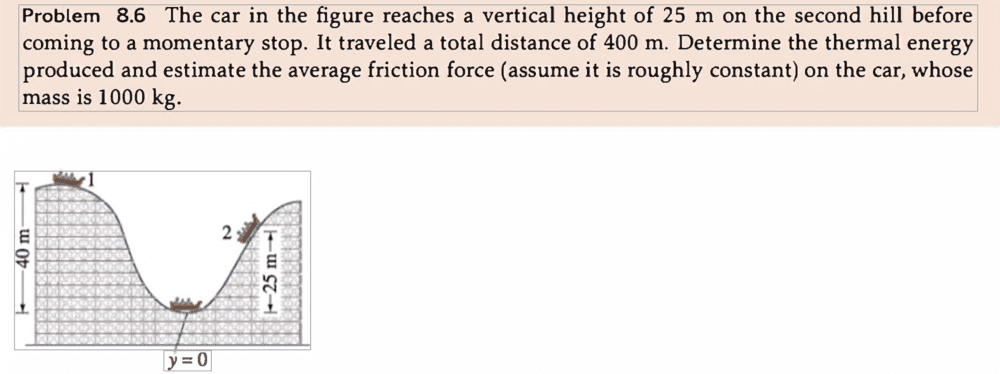# Is Nonconservative work negative?

• guyvsdcsniper
Please continue any discussion of this topic there.In summary, the thermal energy produced in this problem is +147,000J, as determined by the equation Q = -W. This is because friction, which does negative work, has removed 147,000J of mechanical energy and converted it to thermal energy. Non-conservative work is not necessarily negative; it can also be positive in certain situations, such as when a magnetic field does work.f

#### guyvsdcsniper

Homework Statement
The car in the figure reaches a vertical height of 25 m on the second hill before coming to a momentary stop. It traveled a total distance of 400 m. Determine the thermal energy produced and estimate the average friction force (assume it is roughly constant) on the car, whose mass is 1000 kg.
Relevant Equations
Wnet=Wc+WNC
I am a bit confused. My teacher solved this problem and his answer for the thermal energy produced was -147,000J. My book says it is 147000J. My teacher used the equation K2+P2=K1+P1+Wnc and my book used K1+P1=K2+P2+Wnc.

Which one is it?Friction force has opposite direction to velocity of car so
W:work which friction force does on the car has negative sign.
$$W=\int \mathbf{F}\cdot \mathbf{v} dt < 0$$
P1+W=P2 so P1> P2. The car stops at lower position. You observe car energy change.

Q:The thermal energy floor got by giving minus work
Q=-W > 0
P1=P2+Q
You observe car and floor energy conservation.

You may choose to use negative W or positive Q of same amount.

Last edited:
Total energy does not change, ##\Delta E_{total}=0##. From the starting point at height 40 m to the final height of 25 m the only energy changes are gravitational potential, ##\Delta U_{grav}## and thermal, ##\Delta E_{therm}##. Thus, $$0=\Delta E_{total}=\Delta U_{grav}+\Delta E_{therm}~\Rightarrow~\Delta E_{therm}=-\Delta U_{grav}.$$Now $$\Delta U_{grav}=mgh_{\text{f}}-mgh_{\text{i}}=mg(h_{\text{f}}-h_{\text{i}})=1000\times 9.8(-15)~\text{J}=-147,000~\text{J}.$$Thus, ##\Delta E_{therm}=+147,000~\text{J}.## The thermal energy increases which makes sense because the track's and the car's temperatures rise.

Note: Here we considered a three-part closed system consisting of the car the track and the Earth.

Last edited:
I am a bit confused. My teacher solved this problem and his answer for the thermal energy produced was -147,000J. My book says it is 147000J. My teacher used the equation K2+P2=K1+P1+Wnc and my book used K1+P1=K2+P2+Wnc.

Which one is it?
The question specifically asks for the ‘thermal energy produced’ (Q). This will be a positive value.

The work done by friction (W) will be a negative value, because friction and displacement are in opposite directions. A negative value for W tells us that friction has removed mechanical energy.

In this problem: Q = -W.

Friction has removed 147,000J (hence W = -147,000J). What has friction done with this removed energy? - it has converted it to thermal energy, so thermal energy produced = +147,000J.

Personally I would use:
P1 + K1 + W = P2 + K2 (remembering W is negative here and Q = -W)

To answer the question-title: “Is Non-conservative work negative?”
Not necessarily. Friction/resistance forces do negative work because force and displacement are in opposite directions. That’s the most common situation. But (in the right circumstances) a magnetic field is a non-conservative force which can do positive work. I’m sure there are other example of non-conservative work being positive.

EDIT: A discussion (off-topic) about whether a magnetic field can do work (e.g. in an electric motor) followed this post. This has now been moved to a separate thread: https://www.physicsforums.com/threads/magnetic-fields-and-work.1001067/

Last edited: